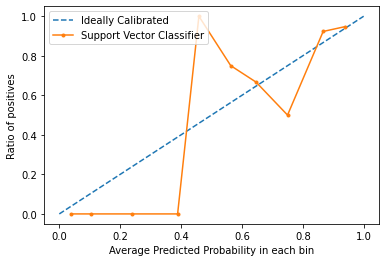Open in App
Not now

# Calibration Curves

• Difficulty Level : Easy
• Last Updated : 14 Sep, 2021

Generally, for any classification problem, we predict the class value that has the highest probability of being the true class label. However, sometimes, we want to predict the probabilities of a data instance belonging to each class label. For example, say we are building a model to classify fruits and we have three class labels: apples, oranges, and bananas (each fruit is one of these). For any fruit, we want the probabilities of the fruit being an apple, an orange, or a banana.
This is very useful for the evaluation of a classification model. It can help us understand how ‘sure’ a model is while predicting a class label and may help us interpret how decisive a classification model is. Generally, classifiers that have a linear probability of predicting each class’s labels are called calibrated. The problem is, not all classification models are calibrated.
Some models can give poor estimates of class probabilities and some do not even support probability prediction.

Calibration Curves:
Calibration curves are used to evaluate how calibrated a classifier is i.e., how the probabilities of predicting each class label differ. The x-axis represents the average predicted probability in each bin. The y-axis is the ratio of positives (the proportion of positive predictions). The curve of the ideal calibrated model is a linear straight line from (0, 0) moving linearly.

Plotting Calibration Curves in Python3:

Code: Implementing a Support Vector Machine’s calibration curve and compare it with a perfectly calibrated model’s curve.

## Python3

 `# Importing required modules` `from` `sklearn.datasets ``import` `load_breast_cancer``from` `sklearn.svm ``import` `SVC``from` `sklearn.model_selection ``import` `train_test_split``from` `sklearn.calibration ``import` `calibration_curve``import` `matplotlib.pyplot as plt` `# Loading dataset``dataset ``=` `load_breast_cancer()``X ``=` `dataset.data``y ``=` `dataset.target` `# Splitting dataset into training and testing sets``X_train, X_test, y_train, y_test ``=` `train_test_split(X, y,``                     ``test_size ``=` `0.1``, random_state ``=` `13``)` `# Creating and fitting model``model ``=` `SVC()``model.fit(X_train, y_train)` `# Predict Probabilities``prob ``=` `model.decision_function(X_test)` `# Creating Calibration Curve``x, y ``=` `calibration_curve(y_test, prob, n_bins ``=` `10``, normalize ``=` `True``)` `# Plot calibration curve` `# Plot perfectly calibrated``plt.plot([``0``, ``1``], [``0``, ``1``], linestyle ``=` `'--'``, label ``=` `'Ideally Calibrated'``)` `# Plot model's calibration curve``plt.plot(y, x, marker ``=` `'.'``, label ``=` `'Support Vector Classifier'``)` `leg ``=` `plt.legend(loc ``=` `'upper left'``)``plt.xlabel(``'Average Predicted Probability in each bin'``)``plt.ylabel(``'Ratio of positives'``)``plt.show()`

Output:From the graph, we can clearly see that the Support Vector classifier is nor very well calibrated. The closes a model’s curve is to the perfect calibrated model’s curve (dotted curve), the better calibrated it is.

Conclusion:
Now that you know what calibration is in terms of Machine Learning and how to plot a calibration curve, next time you classifier gives unpredictable results and you can’t find the cause, try plotting the calibration curve and check if the model is well-calibrated.

My Personal Notes arrow_drop_up